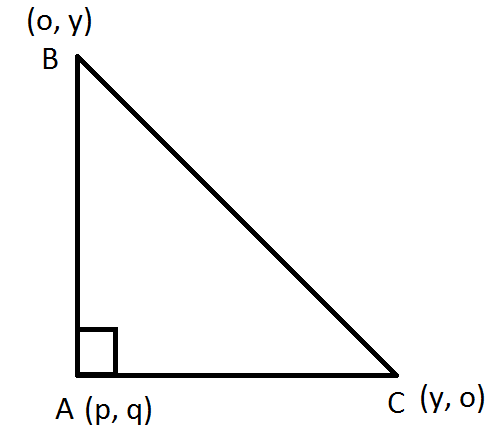QuestionAnswers

# Find the locus of the third vertex of a right-angled triangle, the ends of whose hypotenuse are (4,0) and (0,4).Verified
128.7k+ views
Hint: a locus (Latin word for "place", "location") is a set of all points (commonly, a line, line segment a curve, or a surface) whose location satisfies or is determined by one or more specified conditions. In other words, the set of points that satisfy some property is often called the locus of a point satisfying this property.
The locus of a point P is such that it is equidistant from two given points. A and B i.e. PA=PB. The locus of a point at fixed distance d, from point P, is a circle with given point P as its center and d as its radius. Here in this question, we can use the distance formula for two given points.
Distance formula = $\sqrt {{{(x_2 - x_1)}^2} + {{(y_2 - y_1)}^2}}$Let the point be $(p,q)$
$B{C^2} = A{B^2} + A{C^2}$(By Pythagoras theorem)
${(4 - 0)^2} + {(0 - 4)^2} = {(p - 0)^2} + {(q - 4)^2} + {(p - 4)^2} + {(q - 0)^2}$
$16 + 16 = {p^2} + {q^2} + 16 - 8q + {p^2} + 16 - 8p + {q^2}$
$32 = 2({p^2} + {q^2} - 4p - 4q) + 32$
Or ${p^2} + {q^2} - 4p - 4q = 0$
Replacing $p \to x$and $q \to y$
${x^2} + {y^2} - 4x - 4y = 0$ is the locus of the equation.

Note: we can also find this finding slope of two lines AB as $m_1 = \dfrac{{y_2 - y_1}}{{x_2 - x_1}}$, and AC as $m_2 = \dfrac{{y_2 - y_1}}{{x_2 - x_1}}$
And for perpendicular lines${m_1}{m_2} = - 1$
We get the required locus of the third vertex.